# NCERT solutions for class 9 science chapter 8 Motion

In this page we have NCERT solutions for class 9 science chapter 8 (intext and Exercise) on Motion (Physics) . Hope you like them and do not forget to like , social shar and comment at the end of the page.

## NCERT intext Solutions for Motion

Page 100
Question 1
An object has moved through a distance. Can it have zero displacement? If yes, support your Solution with an example.
Solution
Yes, this is very much possible.
An object can have zero displacement even when it has moved through a distance. This happens when final position of the object coincides with its initial position.
Examples:
(a)A person moves around park and stands on place from where he started then here displacement will be zero but distance travelled will not be zero
(b) If you go around a circle and come back to the starting point, then displacement will be zero but distance will not be

Question 2
A farmer moves along the boundary of a square field of side 10 m in 40 s. What will be the magnitude of displacement of the farmer at the end of 2 minutes 20 seconds from his initial position?
Solution
Let ABCD is the square field and Farmer starts at point A and moves in the anticlockwise direction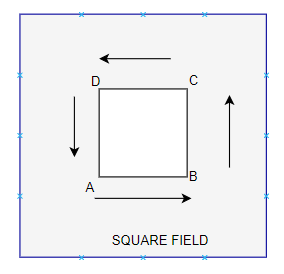Given in the problem
Side of the square field= 10m
Therefore, perimeter = 10 m x 4 = 40 m
Farmer moves along the boundary in 40s.

So speed of the farmer= distance travelled/ Time taken = 40 /40   = 1m/s
Displacement after 2 m 20 s = 2 x 60 s + 20 s = 140 s =?
Distance travelled in 140s =speed × time= 1 × 140 m = 140 m
Now, number of Lap to cover 140 along the boundary= Total Distance / Perimeter
= 140 m / 40 m = 3.5 round
Thus, after 3.5 round farmer will at point C of the field.
So displacement = Magnitude of AC = Diagonal of the square of side 10 m
=10(2)1/2
=14.14m
Important Conceptual Question

Question 3
Which of the following is true for displacement?
(a) It cannot be zero.
(b) Its magnitude is greater than the distance travelled by the object.
Solution
None of the statement is true for displacement as
(1)displacement can be zero.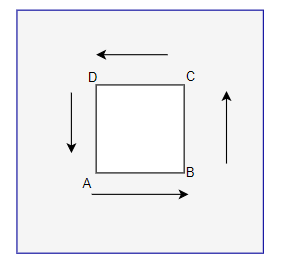In the above figure , A person moves from point A and travelled along the square reach back to A. Its displacement is zero
(2) displacement is less than or equal to the distance travelled by the object.
In the above figure, A person moves from point A to B and then C, Now Displacement is along AC while distance is AB + BC.So displacement magnitude is never greater than the distance travelled by the object

Page 102
Question 4
Distinguish between speed and velocity.
Solution
 Speed Velocity Speed is the distance travelled by an object in a given interval of time. Velocity is the displacement of an object in a given interval of time. Speed = distance / time Velocity = displacement / time Speed is scalar quantity i.e. it has only magnitude. Velocity is vector quantity i.e. it has both magnitude as well as direction.

Question 5
Under what condition(s) is the magnitude of average velocity of an object equal to its average speed?
Solution
The magnitude of average velocity of an object is equal to its average speed, only when an object is moving in a straight line.

Question 6
What does odometer of an automobile measure?
Solution
The distance covered by an automobile.

Question 7
What does the path of an object look like when it is in uniform motion?
Solution
straight line path.

Question 8
During an experiment, a signal from a spaceship reached the ground station in five minutes. What was the distance of the spaceship from the ground station? The signal travels at the speed of light, that is, 3 × 108 m s−1.
Solution
Given
Speed= 3 × 108 m s−1
Time= 5 min = 5 x 60 = 300 secs.
We know that
Distance= Speed x Time
Distance= 3 × 108 m s−1 x 300 secs. = 9 x 1010 m

Page 103
Question 9
When will you say a body is in
(i) uniform acceleration?
(ii) non-uniform acceleration?
Solution
 Uniform acceleration Non-uniform acceleration A body is said to be in uniform acceleration if it travels in a straight line and its velocity increases or decreases by equal amounts in equal intervals of time. A body is said to be in non-uniform acceleration if the rate of change of its velocity is not constant.

Question 10
A bus decreases its speed from 80 km h−1 to 60 km h−1 in 5 s. Find the acceleration of the bus.
Solution
We have to very careful with units while doing the numerical questions.
Here we would convert km/h into m/s
Initial Speed (u) = 80 km/h= (80 ×1000)/3600 m/s = 22.22 m/s
Final Speed (u) = 60 km/h= (60 ×1000)/3600 m/s = 16.66 m/s
Now
Acceleration is given by
= Change in speed/ time taken
= (v-u)/t
=-1.112 m/s2
Here negative sign denotes de-acceleration

Question 11
A train starting from a railway station and moving with uniform acceleration attains a speed 40 km h−1 in 10 minutes. Find its acceleration.
Solution
Given
Initial velocity of the train (u) =0
Final velocity of the train (v)=40 km/h = 11.11 m/s
Time taken(t) =10 min= 600s
Now
Acceleration is given by
= Change in speed/ time taken
= (v-u)/t
= .0185 m/s2
Page 107

Question 12
What is the nature of the distance - 'time graphs for uniform and non-uniform motion of an object?
Solution
 When the motion is uniform, the distance time graph is a straight line with a slope. When the motion is non uniform, the distance time graph is not a straight line. It can be any curve.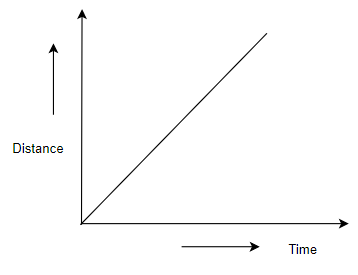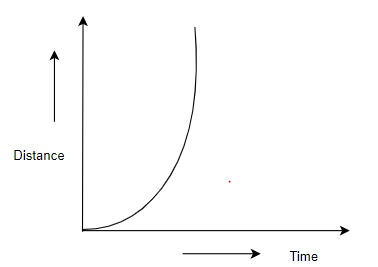Question 13
What can you say about the motion of an object whose distance - time graph is a straight line parallel to the time axis?
Solution
It is at rest

Question 14
What can you say about the motion of an object if its speed - 'time graph is a straight line parallel to the time axis?
Solution
It means speed is constant and object is moving uniformly

Question 15
What is the quantity which is measured by the area occupied below the velocity -time graph?
Solution
The area below velocity-time graph gives the distance covered by the object.

Page 109-110
Question 16
A bus starting from rest moves with a uniform acceleration of 0.1 m /s2 for 2 minutes. Find
(a) the speed acquired
(b) the distance travelled.
Solution
Given
Initial speed of the bus(u) = 0
Acceleration(a) = 0.1 m/s2
Time taken(t) = 2 minutes = 120 s
(a)According the first equation of motion
$v= u+ at$
$v=0 + 0.1 \times 120$
v= 12 m/s
(b)According to the third equation of motion:
$v^2 - u^2= 2as$
Where, s is the distance covered by the bus
$(12)^2 - (0)^2= 2 \times (0.1) \times s$
s = 720 m

Question 17
A train is travelling at a speed of 90 km h−1. Brakes are applied so as to produce a uniform acceleration of −0.5 m s−2. Find how far the train will go before it is brought to rest.
Solution
Initial speed of the train(u)= 90 km/h = 25 m/s
Final speed of the train(v) = 0 (As finally the train comes to rest)
Acceleration = - 0.5 m/s2
According to third equation of motion:
$v^2= u^2+ 2as$
$(0)^2= (25)^2+ 2 \times (- 0.5) \times s$

Where, s is the distance covered by the train
$s = \frac {625}{2(.5)} = 625m$
The train will cover a distance of 625 m before it comes to rest.

Question 18
A trolley, while going down an inclined plane, has an acceleration of 2 cm s−2. What will be its velocity 3 s after the start?
Solution
Given
Initial Velocity of trolley(u)= 0 cm/s
Acceleration(a)= 2 cm /s2
Time, t= 3 s
We know that final velocity is given by Ist equation of motion
$v= u + at$ $v=0 + 2 \times 3$
Therefore, The velocity of train after 3 seconds = 6 cm/s

Question 19
A racing car has a uniform acceleration of 4 m/s2. What distance will it cover in 10 s after start?
Solution
Initial Velocity of the car(u)=0 m/s
Acceleration(a)= 4 m /s2
Time(t)= 10 s
We know Distance is given by 2nd equation of motion
$s= ut + \frac {1}{2} at^2$
Therefore, Distance covered by car in 10 second
$s= 0 \times 10 + \frac {1}{2} \times 4 \times (10)^2$
= 200 m

Question 20
A stone is thrown in a vertically upward direction with a velocity of 5 m/s. If the acceleration of the stone during its motion is 10 m /s2 in the downward direction, what will be the height attained by the stone and how much time will it take to reach there?
Solution
Given
Initial velocity of stone(u)=5 m /s
Acceleration(a)= -10 m /s2(as acceleration is opposite to direction of motion)
Final velocity of stone (v) =0 m/s
We know as per 3rd equation of uniform motion
$2as= v^2- u^2$
$2(-10) s= 0- (5)^2$
s=25/20= 12.5 m
We know as per Ist equation of uniform motion
v=u + at
0=5-10t
t= .5s

## NCERT Exercise Solutions for Motion

Question 21
An athlete completes one round of a circular track of diameter 200 m in 40 s. What will be the distance covered and the displacement at the end of 2 minutes 20 s?
Solution
Given Diameter of circular track (D) = 200 m
Radius of circular track (r) = 200 / 2=100 m
Time taken by the athlete for one round (t) = 40 s
So
Distance covered by athlete in one round (s) = 2π r
= 2 x (22 / 7) x 100 = 4400/7 m
Speed of the athlete (s) = Distance / Time
= (4400) / (7 x 40) = 110/7 m/s
Now Distance covered in 140 s
= Speed × Time
= (110/7) x (140)
= 2200 m

Number of round in 40 s =1 round
Number of round in 140 s =140/40
=3 ½
Let us assume athlete start at point A on the circular ground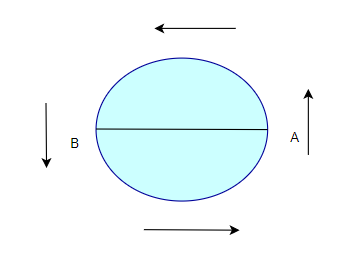After taking start from position A, the athlete will be at position B after 3 1/2 rounds

Hence, Displacement of the athlete with respect to initial position at B= AB

= Diameter of circular track
= 200 m

Question 22
Joseph jogs from one end A to the other end B of a straight 300 m road in 2 minutes 30 seconds and then turns around and jogs 100 m back to point C in another 1 minute. What are Joseph's average speeds and velocities in jogging?
(a) from A to B (b) from A to C?
Solution
Total Distance covered from AB = 300 m
Total time taken = 2 x 60 + 30 s
=150 s
a) Average Speed from AB = Total Distance / Total Time
=300 / 150 m/s
=2 m /s
Average Velocity from AB =Displacement AB / Time = 300 / 150 m s-1
=2 m s-1
b) Total Distance covered from AC =AB + BC
=300 + 100 m
Total time taken from A to C = Time taken for AB + Time taken for BC
=150s+60 s
= 210 s
Average Speed from AC = Total Distance /Total Time
= 400 /210 m /s
= 1.904 m /s
Now Displacement (S) from A to C = AB - BC
= 300-100 m
= 200 m
Time (t) taken for displacement from AC = 210 s
Average Velocity from AC = Displacement (s) / Time(t)
= 200 / 210 m /s
= 0.952 m /s

Question 23
Abdul, while driving to school, computes the average speed for his trip to be 20 km h−1. On his return trip along the same route, there is less traffic and the average speed is 30 km h−1. What is the average speed for Abdul’s trip?
Solution
let the distance Abdul commutes while driving from Home to School = d
Let us assume time taken by Abdul to commutes this distance = t1
Now Distance Abdul commutes while driving from School to Home = d
Let us assume time taken by Abdul to commutes this distance = t2
Average speed from home to school v1 = 20 km/h
Average speed from school to home v2 = 30 km/h
Now
we know Time taken from Home to School t1 =d/ v1 = d/20
Similarly Time taken from School to Home t2 =d/v2 = d/30
Total distance from home to school and backward = 2d

Total time taken from home to school and backward (T) = d/20+ d/30
Average speed (Vav) for covering total distance (2S) = Total Distance/Total Time
= 2d / (d/20 +d/60)
= 2d / [(2d+d)/60]
= 120d / 5d
= 24 km/h

Question 24
A motorboat starting from rest on a lake accelerates in a straight line at a constant rate of 3.0 m /s2 for 8.0 s. How far does the boat travel during this time?
Solution
Given

Initial velocity of motor boat(u) = 0
Acceleration of motor boat, a = 3.0 m s-2
Time under consideration, t = 8.0 s
We know that Distance travelled as per 3rd law of uniform motion
$s = ut + \frac {1}{2}at^2$
The distance travel by motorboat
$s= 0 \times 8 + \frac {1}{2} \times 3.0 \times (8)^2$
$= \frac {1}{2} \times 3.0 \times (8)^2$
= 96 m

Question 25
A driver of a car travelling at 52 km h−1 applies the brakes and accelerates uniformly in the opposite direction. The car stops in 5 s. Another driver going at 3 km h−1 in another car applies his brakes slowly and stops in 10 s. On the same graph paper, plot the speed versus time graphs for the two cars. Which of the two cars travelled farther after the brakes were applied?
Solution
First driver car Initial speed (uA) = 52km/h = 52 x 1000/3600 = 14.4 m/s
Final Speed (vA) = 0 m/s
Time taken (tA) = 5s

Second driver car Initial speed (uB) = 3km/h = 3 x 1000/3600 = 0.83 m/s
Final Speed (vB) = 0 m/s
Time taken (tB) = 10s

Plotting on Speed-time graph, the graph PR and SQ are the Speed-time graph for given two cars with initial speeds 14.4 m/s and .83 m/s respectively.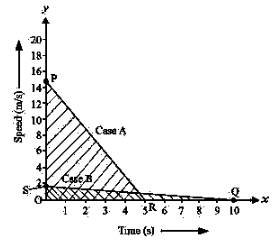Distance Travelled by first car before coming to rest =Area of △ OPR
= (1/2) x OR x OP
= (1/2) x 5 s x 14.4 m/s
= 36.11 m
Distance Travelled by second car before coming to rest =Area of △ OSQ
= (1/2) x OQ x OS
= (1/2) x 10 s x .83 m/s= 4.16 m

Question 26
Below Figure shows the distance-time graph of three objects A, B and C. Study the graph and Solution the following questions: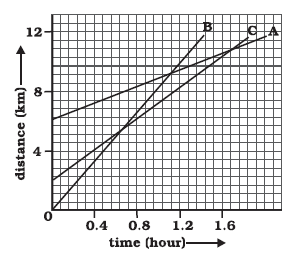(a) Which of the three is travelling the fastest?
(b) Are all three ever at the same point on the road?
(c) How far has C travelled when B passes A?
(d)How far has B travelled by the time it passes C?
Solution
Important points to note
Speed is given by = Distance /time   =  Y-axis/X axis = Slope
So the object with steepest slope will have fastest speed
(a) Object B   Since slope of object B is greater than objects A and C, it is travelling the fastest.
(b) No  as all the three lines never intersect at the same point
c) When B and A meets at point, the Object C is at a point, which is approximately 8km on distance axis.
(d) B meets C   at point . The position on distance-axis is 9 units away from origin O. One unit   on distance-axis = 4/7 (7 units = 4km).
So Object B is = 9 x 4/7 = 5.14 km.

Question 27
A ball is gently dropped from a height of 20 m. If its velocity increases uniformly at the rate of 10 m s−2, with what velocity will it strike the ground? After what time will it strike the ground?
Solution
Initial Velocity of ball(u) =0
Distance or height of fall(s) =20 m
Downward acceleration, a =10 m /s2
As we know from 3rd equation of uniform motion
2as =v2-u2
v2 = 2as+ u2
= 400
So
Final velocity of ball, v = 20 m/s
As we know from 1st equation of uniform motion
$v=u+at$
$t = \frac {v-u}{a}$
Time taken by the ball to strike = (20-0)/10
= 20/10
= 2 seconds

Question 28
The speed-time graph for a car is shown is given below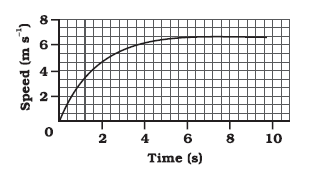(a) Find out how far the car travels in the first 4 seconds. Shade the area on the graph that represents the distance travelled by the car during the period.
(b) Which part of the graph represents uniform motion of the car?
Solution

(a)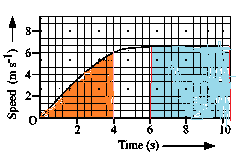The shaded area in orange which is equal to 1 / 2 x 4 x 6 = 12 m represents the distance travelled by the car in the first 4 s.
(b)The part of the graph in blue colour between time 6 s to 10 s represents uniform motion of the car.

Question 29
State which of the following situations are possible and give an example for each of these:
(a) an object with a constant acceleration but with zero velocity.
(b) an object moving in a certain direction with an acceleration in the perpendicular direction.
Solution
(a) Possible
When a ball is thrown up at maximum height, it has zero velocity, although it will have constant acceleration due to gravity, which is equal to 9.8 m/s2

(b) Possible
When a car is moving in a circular track, its acceleration is perpendicular to its direction.

Question 30
An artificial satellite is moving in a circular orbit of radius 42250 km. Calculate its speed if it takes 24 hours to revolve around the earth.
Solution
Radius of the circular orbit, r= 42250 km
Time taken to revolve around the earth, t= 24 h
Speed of a circular moving object, v= (2π r)/t
=3073.74 m /s

## Summary

1. NCERT (intext and Exercise) Solutions on Motion for Class 9 physics has been prepared by Expert with utmost care. If you find any mistake.Please do provide feedback on mail. You can download the solutions as PDF in the below Link also